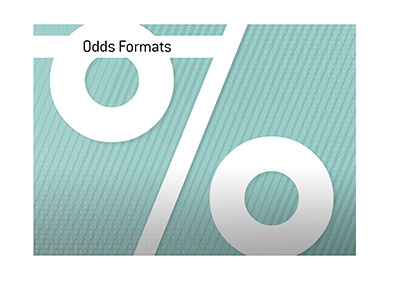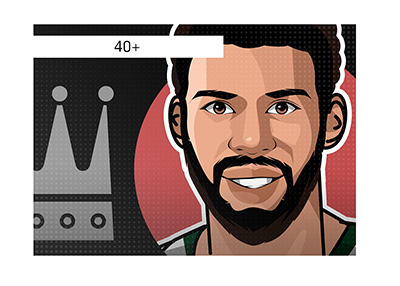# A Look at the Different Odds Formats And How To Calculate Your Profit

Published on January 5th, 2021 7:21 pm EST
Written By: Dave ManuelSportsbooks use different odds formats.

You have decimal odds, fractional odds and American odds.

All of these odds formats boil down to the same thing - they are simply a different way of presenting the information to you.

One of the most frequently asked questions that we see in the sports betting world: how do I calculate my potential winnings using each of the different odds formats?

Here is a quick and easy way of figuring out your potential profit for all three.

Decimal Odds

This is probably the easiest odds format to calculate.

Let's say that the odds for a fighter that you want to bet on look like this:

1.80

In order to calculate your potential profit, simply multiply this number by your wager, and then subtract the amount of your wager to get your profit.

So, if you bet \$100 on this fighter at 1.80, your calculation would look like this:

\$100 * 1.80 = \$180

\$180 - \$100 = \$80

You'd be looking at a profit of \$80.

If the odds were something like 3.60, it would look like this:

\$100 * 3.60 = \$360

\$360 - \$100 = \$260

Your potential profit in this case would be \$260

American Odds

With American odds, you will see a number with either a + or - next to it.

For instance, the number might look like this:

+200

Or like this:

-330

Here is how you calculate the potential profit for either one:

In the case of a number with a + in front of it (which means that the side is an underdog), the amount of the number is the amount of profit that you would earn with a bet of \$100.

So, if you bet \$100 on a +200 line, you would realize a potential profit of \$200.

So, your potential profit would be 2x your original bet. So, if you were betting \$20, your potential profit would be \$40, and so on.

With a line like -330, this means that side is an underdog.

This means that you would need to wager \$330 in order to have a chance of winning \$100 in profit.

So, simply divide \$100 by \$330 and then multiply this number by your bet size in order to see how much profit you could earn.

So, if you bet \$50, your potential profit would be a little over \$15.

Fractional Odds

Let's say that you have a number that looks like this:

3/2

In order to figure out your potential profit, divide 3 by 2 and then multiply this number by your bet to find your potential profit.

So, in this case, we divide 3/2 to get 1.5.

We then multiply this number by our bet (let's say \$100) to get \$150, which represents the amount of our potential profit on this bet.

-

That's it!

Sportsbooks will almost always let you choose your odds format of choice, and there are plenty of calculators available online to help you figure out the odds, including this one.

#### Related Articles### The Time Tiger Woods Shot a Front Nine 28 At The 2007 Tour Championship

In 2007, Tiger Woods was at the height of his powers, free of health and personal issues. Woods ...### What Are the Five Most Impressive Seasons for a 40+ Year-Old Athlete Ever?

Gordie Howe. Kareem Abdul-Jabbar. Ted Williams. Nolan Ryan. Tom Brady. What do these five men ha...### Would You Bet on a WWE Match?

Would you bet on the outcome of a scripted WWE (World Wrestling Entertainment, Inc.) match? Accor...